CO2 Use in the Fischer-Tropsch Process

Emmanuel Bonifacio November 29, 2010

Submitted as coursework for Physics 240, Stanford University, Fall 2010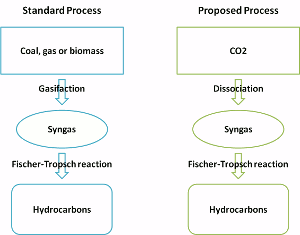Fig. 1: The new proposed Fischer-Tropsch process

CO2 dissociation into carbon and oxygen is the mechanism that plants use to fuel their growth. One could think that an analogous process could be devised in order to generate fuel from CO2, potentially using renewable energy sources to drive the process. This would potentially yield a source of hydrocarbon fuels from the abundant (and increasing) supply of CO2, providing both a means of control on CO2 emissions as well as a means of production for hydrocarbon fuels once geologic supplies are depleted.

Reactions

Such a mechanism can be elaborated by combining two well studied reactions. We can see that if we couple the following two reactions:

1. CO2 <=> CO + 1/2 O2
2. (2n+1) H2 + n CO2 <=> H2n+2 + n H2O

where (1) is the dissociation of CO2 in carbon monoxide and oxygen and and (2) is the Fischer-Tropsch process, we will be able to produce hydrocarbons from CO2. This is equivalent to declaring that the carbon source in our Fischer-Tropsch process will be carbon dioxide instead of the usual gasified coal methane or bio-mass.

The Fischer-Tropsch process was originally developed in the 1920s and used on an industrial by Germany during World War II to ensure continuous supply of fuel from coal. Coal was first gasified into a mixture of carbon monoxide and hydrogen, called syngas then catalyzed through the process into hydrocarbon chains of varying length. One must note that the catalyst itself plays a major role in determining the length of the hydrocarbon chain and the speed and efficiency of the reaction.  This process does not, of course, create energy, and requires heating of the syngas, the heat being transferred into chemical energy through the bonding of the hydrocarbon chains.

Energy Requirements

The carbon dioxide dissociation reaction is however the main hurdle in this process. Indeed carbon dioxide is very stable under usual temperature and pressure conditions, and this is why so much energy can be extracted by burning fuels into carbon dioxide. Thus large inputs of energy (for example in the form of heat) would be required for reaction (1) to take place. The amount of energy required can be calculated if one considers all the energy is provided through heating. In order for the reaction to be favored to the right, one must increase the equilibrium constant, which is a function of temperature: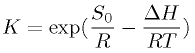where S0 and ΔH0 can be derived thanks to tabulated values at standard temperature and pressure.  One must note that this equation is an approximation as both S0 and ΔH0 may vary with temperature, but we will neglect these variations for the purpose of this report. For our reaction we find that S0=86.5 J/mol-K and ΔH0=283.5 kJ/mol under standard temperature and pressure.

If we consider the reaction of 1 mol of CO2 and we call x the fraction of CO at equilibrium then by definition of the equilibrium constant we have: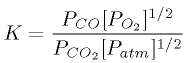Since we know from the reaction that PCO=x/(1 + x/2) P and PO2=x/2/(1 + x/2) P and PCO2=(1-x)/(1+x/2) P we see that this equation boils down to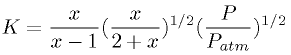Thus if we know the pressure and temperature under which we operate, we can determine K then x. With a sufficient x, one could expect a consequent driving force for separation of CO from the mixture (through a membrane for example) and could thus perpetrate dissociation of incoming CO2. Of course the rate of dissociation of CO2 would raise with the temperature the amount of CO extracted from the mixture and the amount of CO2 feed into the reactor.

We find that if we want x to be around 0.5 at equilibrium, this would yield a mixture with a little less than 50% CO which should be readily extractable, we need to increase the temperature to 3050 K under atmospheric pressure. Knowing the CO2 calorific capacity (37.1 J/mol-K), we find that the required amount of energy for the dissociation reaction to take place is 102.1 kJ/mol of CO2 in the reactor.  This means 204.2 kJ/mol of CO produced. Knowing that the molar weight of CO is 28 g/mol we can see that the required energy is 7.29 x 106 J/kg of CO produced.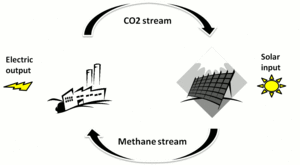Fig. 2: The carbon neutral cycle

Through the Fischer-Tropsch process, we can then catalyze this CO in hydrocarbons. Assuming one would be able to generate this stream of hydrocarbons at peak daylight hours with solar energy, the produced fuels could then be used during the rest of the day as the raw input for base load power plants. CO2 could then be captured at the plant's emissions stream and cycled in back to the solar plant. Thus one would generate a clean continuous source of power.

Carbon Cycle

For example let us assume that we want to power a 10MW base load plant continuously during the day through that process. If we consider a Methane powered plant, with a combined gas turbine reaching an efficiency of 60% in energy conversion, we can determine the amount of gas that must be burnt to keep the plant running. We know that the lower heating value (amount of energy that can be recovered when burning the methane) of a mol of methane is 802.34 kJ/mol thus we find that we need to burn 2.08 mol/s of methane in order to generate the required 10 MW. This means that for a complete combustion, we will in turn produce 2.08 mol/s of CO2. This CO2 can be separated through a CO2 capture unit that works off the plant's power supply. This would in theory necessitate an additional amount of methane input but we will neglect this factor here.

Thus our CO2 based Fischer-Tropsch process should be able to provide 2.08 mol/s of methane over the whole course of the day. What's more, it is of no interest to have this process run off fossil fuels as we could more efficiently use them directly in powering the plant. Thus we should consider renewable energy sources to run the process and, for this report, we will look at solar energy. Let us assuming that the Fischer-Tropsch process has a conversion rate of 1 mol of methane per mol of CO inputted. This is unrealistic, but detailed considerations of the conversion rate require accurate knowledge of the exact operating conditions, we must however note that our CO should be cooled down, but not to low temperatures, as CH4 selectivity rises with increasing temperature. 

Given this assumption, our total cycle is carbon balanced, as CO2 is converted to CO to methane and back to CO2. Thus we know that our required energy is that of heating 2.08 mol/s of CO2 to our chosen dissociation reaction temperature of 3050. In practice detailed considerations on the impact of temperature on the reaction's speed would enable a choice ensuring that 2.08 mol of CO can be extracted per second.

Considering our previous considerations, since we need 204.2 kJ/mol of CO extracted (neglecting the cost of separation) we find that our solar plant must generate 424.7 kW. If we consider a 20% efficient solar energy capture device, and considering a 5.78 kWh/m2/day averaged incident solar power (near Phoenix) we find that the energy could be extracted from an 9000 m2 area.  Of course this solar energy is an average over the course of the day. Thus more energy would be available, and more methane than necessary produced, during the brightest hours and both CO2 emitted by the base load plant during nighttime, as well as methane produced in excess during peak solar hours would need to be stored adequately.

Conclusion

We have found that it is possible, on a reasonable scale, to use CO2 in a Fischer-Tropsch reaction to produce hydrocarbons in order to power a base load power plant. This could result in a closed carbon cycle, enabling continuous power generation from solar power, thanks to storage of gases such as methane or CO2 with reduced greenhouse gas emissions. We have seen that the required amount of solar panels is reasonable, although we must acknowledge the fact that extraction and transport of the CO2 from the gas fired plant as well as extraction of CO from the CO2 dissociation mixture and its subsequent cooling would induce higher energetic needs. One must also not forget the energy required for the production of hydrogen to fuel the Fischer-Tropsch catalytic process.

© Emmanuel Bonifacio. The author grants permission to copy, distribute and display this work in unaltered form, with attribution to the author, for noncommercial purposes only. All other rights, including commercial rights, are reserved to the author.

References

 M. E. Dry, "The Fischer-Tropsch Process: 1950-2000," Catalysis Today 71, 227 (2002).

 NIST Chemistry Webbook, National Institute of Standards and Technology, 2008.

 W. M. Haynes, Ed, CRC Handbook of Chemistry and Physics, 91st Edition (CRC Press, 2010).

 W. Marion and S. Wilcox, "Solar Radiation Data Manual for Flat-Plate and Concentrating Collectors," (Red Book), National Renewable Energy Laboratory.